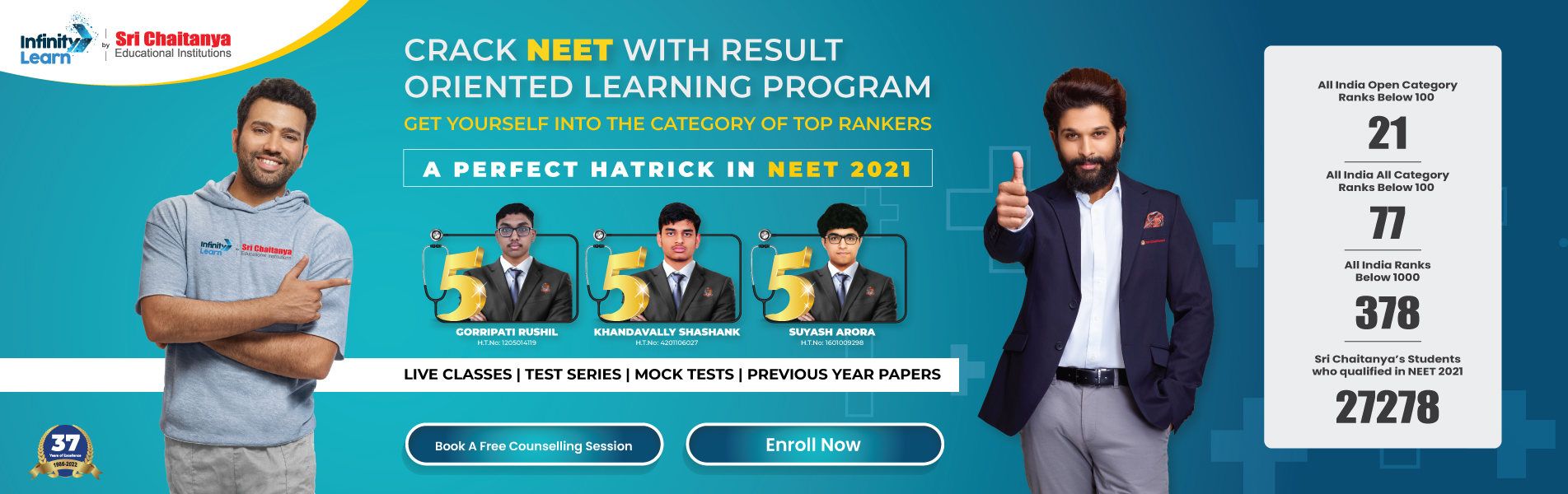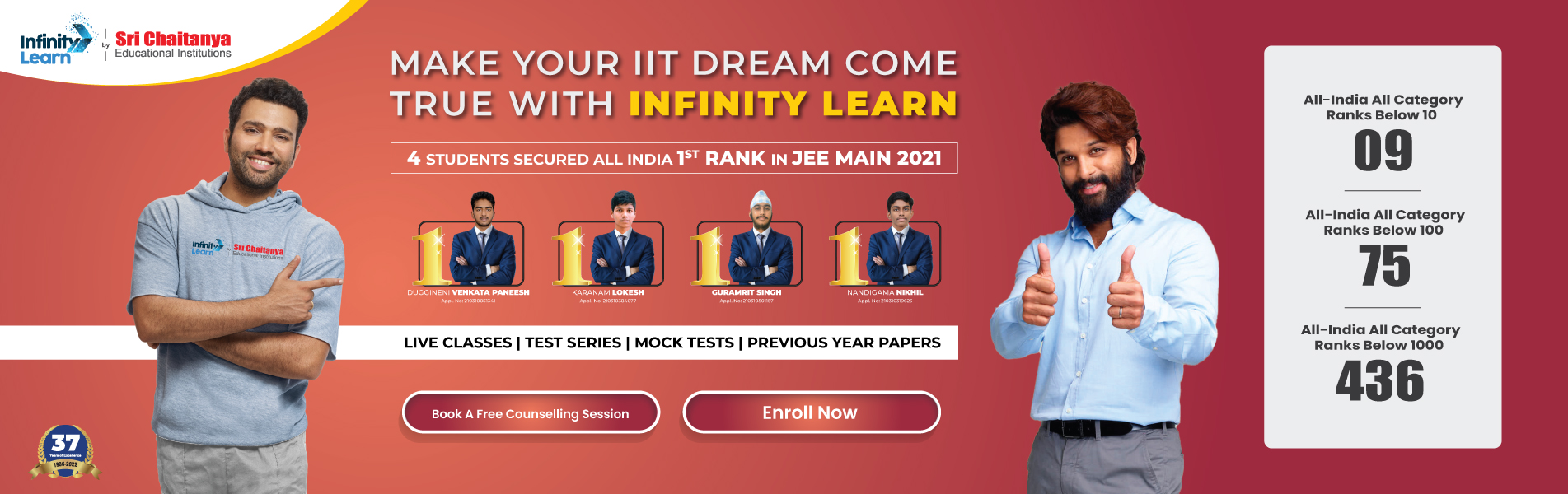NCERT Solutions for Class 6 Maths Chapter 6 – Integers

# NCERT Solutions for Class 6 Maths Chapter 6 – IntegersInfinity Learn NEET IIT-JEE
Infinity Learn NEET
banner-chaitanya-web

Subject specialists have designed NCERT solutions for Maths Class 6 Chapter 6 which includes thorough solutions for reference. These solutions are updated according to the latest CBSE syllabus for 2012-22 and are provided in easy language for understanding. Tips and tricks are also provided.

These solutions are provided so a student can clear his doubts and get help with a deep understanding of the concept. Also, you can refer them to make the chapter notes and revisions notes. PDF of this can also be downloaded from the website.

Free IIT-JEE/NEET/NCERT/CBSE Material

Download 100,000+ FREE PDFs, solved questions, Previous Year Papers, Quizzes, and Puzzles directly to your Whatsapp account!

Solutions

NCERT Solutions for Class 6 Maths Chapter 6 – Integers

NCERT solutions for this chapter is the most important study material for those aiming to score high in the exams. This solution explains each and every concept in a straightforward manner, and help students to learn a topic quickly. By this way, understanding the basics and advanced concepts becomes easy. These solutions are also available online for free, and are easily accessible on the website.

NCERT Class 6 Maths Solutions Topic-Wise Discussion

Class 6 Maths Chapter 6 comprise a total of four sections and following is a topic-wise discussion on each of them.

1. Introduction

This chapter start with an introduction that includes some examples. It tells about a girl named Sita who needs 10 bananas for her picnic, but her mother has only 8 of them. Hence, she borrows two from her neighbour. This example further discusses how Sita needs to return 2 bananas to her neighbour. Few other examples like this present in this chapter introduce the concept of integers to students.

2. Integers

The second section of this chapter includes the discussion of Integers class 6. Students already have a knowledge about the natural number; in this chapter, they came to know about concept of negative integers.

Students must learnt the concept of how to add Integer, as it is an important concept for their exam as well as for their daily life’s. They study how to count integers by moving up and down the stairs.

4. Subtraction of Integers With The Help of A Number Line

Following the addition, students also learn how to subtract integers using a single line. It includes different methods to this process, which are further explained.

All Exercises in the Chapter are given below:

– 10 Questions with Solutions

– 5 Questions with Solutions

– 4 Questions with Solutions

Key Features of NCERT Solutions for this chapter

1. NCERT Solutions helps in providing fully resolved step by step solutions to all textbook questions.
2. Set of solutions which comes with a list of all important formulas on algebraic identities.
3. These solutions are made accordance to the latest syllabus.
4. Solutions are prepared by subject matter experts.
5. NCERT Solutions provides help for the preparation of competitive exams.

Frequently Asked Questions on NCERT Solutions of this Chapter

2. What are the benefits of using NCERT solutions?
3. Why should we download NCERT Solutions for Class 6 Maths Chapter 6 from Infinite learn?

NCERT solutions are available online. Students can also download them for free from infinite learn. These solution PDFs are absolutely free as they are very helpful for board examinations. Infinite learn is a reputed website from where students can download NCERT solutions of any class and chapter.

2. What are the benefits of using NCERT solutions?

NCERT solutions by Infinite Learn is designed strictly according to the latest CBSE syllabus and it is the best way to study this chapter containing all the important points and formulas. The subject matter experts designed these NCERT solutions with an aim to make the learning process a most convenient way.

3. Why should we download NCERT Solutions for Class 6 Maths Chapter 6 from Infinite learn?

At INFINITE LEARN, you can get PDF of accurate solutions of this chapter. The NCERT Textbook Solutions for this chapter have been formulated by mathematics experts at INFINITE LEARN. All these solutions are accordance to the new pattern of CBSE, so the students can be ready for the exams.

Join Infinity Learn Regular Class Programme!